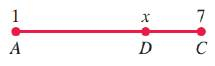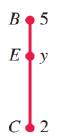# Now suppose that we want to find the coordinates of point P , which is located two-thirds of the distance from A ( 1 , 2 ) to B ( 7 , 5 ) in a coordinate plane. We have plotted the given points A and B in Figure 7.36 to help with the analysis of this problem. Point D is two-thirds of the distance from A to C because parallel lines cut off proportional segments on every transversal that intersects the lines. Figure 7.36 Thus A C ¯ can be treated as a segment of a number line, as shown in Figure 7.37. Therefore, x = 1 + 2 3 ( 7 − 1 ) = 1 + 2 3 ( 6 ) = 5 Figure 7.37 Similarly, C B ¯ can be treated as a segment of a number line, as shown in Figure 7.38. Therefore, y = 2 + 2 3 ( 5 − 2 ) = 2 + 2 3 ( 3 ) = 4 The coordinates of the point P are ( 5 , 4 ) . Figure 7.38 For each of the following, find the coordinates of the indicated point in the x y plane. (a) One-third of the distance from ( 2 , 3 ) to ( 5 , 9 ) (b) Two-thirds of the distance from ( 1 , 4 ) to ( 7 , 13 ) (c) Two-fifths of the distance from ( − 2 , 1 ) to ( 8 , 11 ) (d) Three-fifths of the distance from ( 2 , − 3 ) to ( − 3 , 8 ) (e) Five-eighths of the distance from ( − 1 , − 2 ) to ( 4 , − 10 ) (f) Seven-eighths of the distance from ( − 2 , 3 ) to ( − 1 , − 9 )### Intermediate Algebra

10th Edition
Jerome E. Kaufmann + 1 other
Publisher: Cengage Learning
ISBN: 9781285195728

#### Solutions

Chapter
Section### Intermediate Algebra

10th Edition
Jerome E. Kaufmann + 1 other
Publisher: Cengage Learning
ISBN: 9781285195728
Chapter 7.3, Problem 69PS
Textbook Problem
1 views

## Now suppose that we want to find the coordinates of point P, which is located two-thirds of the distance from A ( 1 , 2 ) to B ( 7 , 5 ) in a coordinate plane. We have plotted the given points A and B in Figure 7.36 to help with the analysis of this problem. Point D is two-thirds of the distance from A to C because parallel lines cut off proportional segments on every transversal that intersects the lines.Figure 7.36Thus A C ¯ can be treated as a segment of a number line, as shown in Figure 7.37. Therefore, x = 1 + 2 3 ( 7 − 1 ) = 1 + 2 3 ( 6 ) = 5Figure 7.37Similarly, C B ¯ can be treated as a segment of a number line, as shown in Figure 7.38. Therefore, y = 2 + 2 3 ( 5 − 2 ) = 2 + 2 3 ( 3 ) = 4 The coordinates of the point P are ( 5 , 4 ) .Figure 7.38For each of the following, find the coordinates of the indicated point in the x y plane.(a) One-third of the distance from ( 2 , 3 ) to ( 5 , 9 ) (b) Two-thirds of the distance from ( 1 , 4 ) to ( 7 , 13 ) (c) Two-fifths of the distance from ( − 2 , 1 ) to ( 8 , 11 ) (d) Three-fifths of the distance from ( 2 , − 3 ) to ( − 3 , 8 ) (e) Five-eighths of the distance from ( − 1 , − 2 ) to ( 4 , − 10 ) (f) Seven-eighths of the distance from ( − 2 , 3 ) to ( − 1 , − 9 )

(a)

To determine

To find:

The coordinates of the point in the xy plane.

### Explanation of Solution

Given:

The given information is that the two points are (2,3) and (5,9).

The required distance is one-third of the given points,

Calculation:

From the given information, the two points are (2,3) and (5,9).

The required distance is 13 of the distance between (2,3) and (5,9).

Let the required coordinates be (x,y).

The value of x is given by,

(b)

To determine

To find:

The coordinates of the point in the xy plane.

(c)

To determine

To find:

The coordinates of the point in the xy plane.

(d)

To determine

To find:

The coordinates of the point in the xy plane.

(e)

To determine

To find:

The coordinates of the point in the xy plane.

(f)

To determine

To find:

The coordinates of the point in the xy plane.

### Still sussing out bartleby?

Check out a sample textbook solution.

See a sample solution

#### The Solution to Your Study Problems

Bartleby provides explanations to thousands of textbook problems written by our experts, many with advanced degrees!

Get Started

Find more solutions based on key concepts
Use the definition of the derivative to find the slope of the tangent line to the graph of the function f(x) = ...

Applied Calculus for the Managerial, Life, and Social Sciences: A Brief Approach

Prove the identity. 15. sinh 2x = 2 sinh x cosh x

Single Variable Calculus: Early Transcendentals, Volume I

In Exercises 1728, use the logarithm identities to obtain the missing quantity.

Finite Mathematics and Applied Calculus (MindTap Course List)

Find the sum of the series. n=0(1)n13nn5n

Calculus (MindTap Course List)

Evaluate the integral. 35. /2/2x1+cos2xdx

Calculus: Early Transcendentals

In words, explain what is measured by SS, variance, and standard deviation.

Statistics for The Behavioral Sciences (MindTap Course List)

Verify each identity. cotx=sin4x+sin6xcos4xcos6x

Trigonometry (MindTap Course List)

Find the limit or show that it does not exist. limtttt2t3/2+3t5

Single Variable Calculus: Early Transcendentals

True or False: .

Study Guide for Stewart's Single Variable Calculus: Early Transcendentals, 8th

The vector represented by where A(4, 8) and B(6, 6)is:

Study Guide for Stewart's Multivariable Calculus, 8th

Express each value as a percent. 25. 1732

Mathematics For Machine Technology